## Example Questions

### Example Question #5 : How To Find Out When An Equation Has No Solution

Find the solution to the following equation if x = 3:

y = (4x2 - 2)/(9 - x2)

0

3

6

no possible solution

no possible solution

Explanation:

Substituting 3 in for x, you will get 0 in the denominator of the fraction. It is not possible to have 0 be the denominator for a fraction so there is no possible solution to this equation.

### Example Question #9 : How To Find Out When An Equation Has No Solution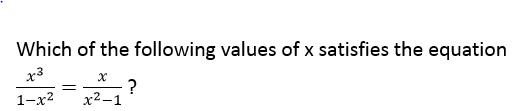I.  x = 0

II. x = –1

III. x = 1

I only

II and III only

I, II, and III

III only

II only

I only

Explanation: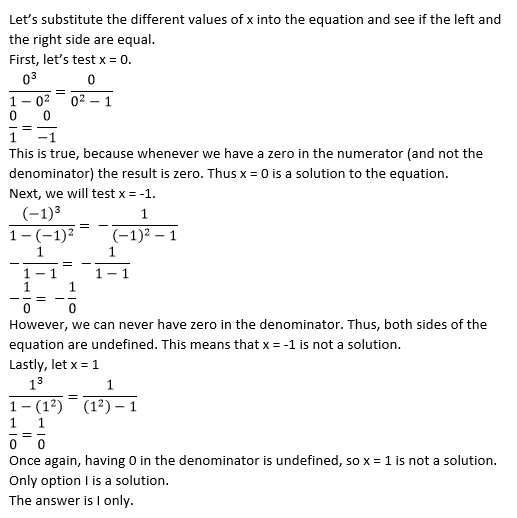### Example Question #6 : How To Find Out When An Equation Has No Solution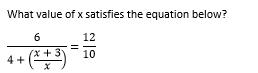–1/2

3

–3

There is no solution

1

There is no solution

Explanation: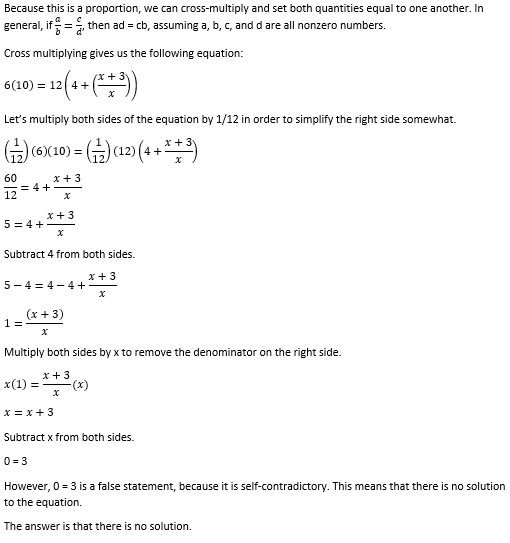### Example Question #7 : How To Find Out When An Equation Has No Solution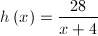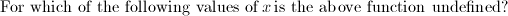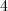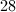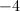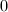Explanation:

A fraction is considered undefined when the denominator equals 0. Set the denominator equal to zero and solve for the variable.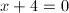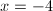### Example Question #10 : How To Find Out When An Equation Has No Solution

Solve: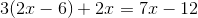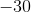Explanation:

First, distribute, making sure to watch for negatives.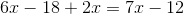Combine like terms.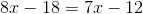Subtract 7x from both sides.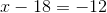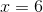### Example Question #3 : How To Find Out When An Equation Has No Solution

Solve: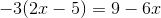Infinitely Many Solutions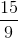No Solution

No Solution

Explanation:

First, distribute theto the terms inside the parentheses.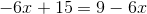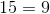This is false for any value of. Thus, there is no solution.

### Example Question #6 : Linear / Rational / Variable Equations

Solve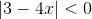.

No solutions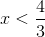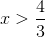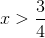No solutions

Explanation:

By definition, the absolute value of an expression can never be less than 0. Therefore, there are no solutions to the above expression.

### Example Question #1 : How To Find Out When An Equation Has No Solution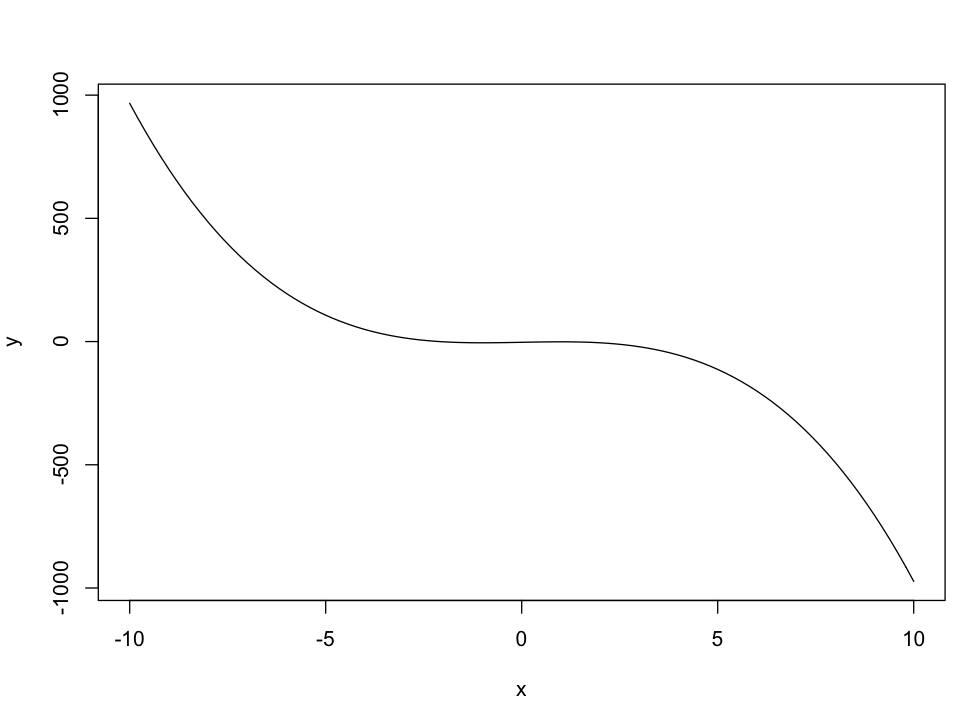In the above graphic, approximately determine the x values where the graph is neither increasing or decreasing.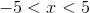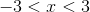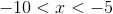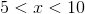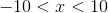We need to find where the graph's slope is approximately zero. There is a straight line between the x values of, and. The other x values have a slope. So our final answer is.